• 常用的几种排序算法
千次阅读
2021-06-08 14:07:30

# 1.常见算法分类

十种常见排序算法一般分为以下几种：
（1）非线性时间比较类排序：交换类排序（快速排序和冒泡排序）、插入类排序（简单插入排序和希尔排序）、选择类排序（简单选择排序和堆排序）、归并排序（二路归并排序和多路归并排序）；

（2）线性时间非比较类排序：计数排序、基数排序和桶排序。

总结：
（1）在比较类排序中，归并排序号称最快，其次是快速排序和堆排序，两者不相伯仲，但是有一点需要注意，数据初始排序状态对堆排序不会产生太大的影响，而快速排序却恰恰相反。

（2）线性时间非比较类排序一般要优于非线性时间比较类排序，但前者对待排序元素的要求较为严格，比如计数排序要求待排序数的最大值不能太大，桶排序要求元素按照hash分桶后桶内元素的数量要均匀。线性时间非比较类排序的典型特点是以空间换时间。

# 2.算法描述与实现

## 2.1交换类排序

交换排序的基本方法是：两两比较待排序记录的排序码，交换不满足顺序要求的偶对，直到全部满足位置。常见的冒泡排序和快速排序就属于交换类排序。

### 2.1.1冒泡排序

算法思想：
从数组中第一个数开始，依次遍历数组中的每一个数，通过相邻比较交换，每一轮循环下来找出剩余未排序数的中的最大数并”冒泡”至数列的顶端。

算法步骤：
（1）从数组中第一个数开始，依次与下一个数比较并次交换比自己小的数，直到最后一个数。如果发生交换，则继续下面的步骤，如果未发生交换，则数组有序，排序结束，此时时间复杂度为O(n)；
（2）每一轮”冒泡”结束后，最大的数将出现在乱序数列的最后一位。重复步骤（1）。

稳定性：稳定排序。

时间复杂度： O(n)至O(n2)，平均时间复杂度为O(n2)。

最好的情况：如果待排序数据序列为正序，则一趟冒泡就可完成排序，排序码的比较次数为n-1次，且没有移动，时间复杂度为O(n)。

最坏的情况：如果待排序数据序列为逆序，则冒泡排序需要n-1次趟起泡，每趟进行n-i次排序码的比较和移动，即比较和移动次数均达到最大值：
比较次数:Cmax=∑i=1n−1(n−i)=n(n−1)/2=O(n2)
移动次数等于比较次数，因此最坏时间复杂度为O(n2)。

示例代码：

void bubbleSort(int array[],int len){

//循环的次数为数组长度减一，剩下的一个数不需要排序

for(int i=0;i<len-1;++i){

bool noswap=true;

//循环次数为待排序数第一位数冒泡至最高位的比较次数

for(int j=0;j<len-i-1;++j){

if(array[j]>array[j+1]){

array[j]=array[j]+array[j+1];

array[j+1]=array[j]-array[j+1];

array[j]=array[j]-array[j+1];

//交换或者使用如下方式

//a=a^b;

//b=b^a;

//a=a^b;

noswap=false;

}

}

if(noswap) break;

}

}

### 2.1.2快速排序

冒泡排序是在相邻的两个记录进行比较和交换，每次交换只能上移或下移一个位置，导致总的比较与移动次数较多。快速排序又称分区交换排序，是对冒泡排序的改进，快速排序采用的思想是分治思想。。

算法原理：
(1)从待排序的n个记录中任意选取一个记录（通常选取第一个记录）为分区标准;

(2)把所有小于该排序列的记录移动到左边，把所有大于该排序码的记录移动到右边，中间放所选记录，称之为第一趟排序；

(3)然后对前后两个子序列分别重复上述过程，直到所有记录都排好序。

稳定性：不稳定排序。

时间复杂度： O（nlog2n）至O(n2)，平均时间复杂度为O（nlgn）。

最好的情况：是每趟排序结束后，每次划分使两个子文件的长度大致相等，时间复杂度为O（nlog2n）。

最坏的情况：是待排序记录已经排好序，第一趟经过n-1次比较后第一个记录保持位置不变，并得到一个n-1个元素的子记录；第二趟经过n-2次比较，将第二个记录定位在原来的位置上，并得到一个包括n-2个记录的子文件，依次类推，这样总的比较次数是：

Cmax=∑i=1n−1(n−i)=n(n−1)/2=O(n2)

示例代码：

//a：待排序数组，low：最低位的下标，high：最高位的下标

void quickSort(int a[],int low, int high)

{

if(low>=high)

{

return;

}

int left=low;

int right=high;

int key=a[left]; /*用数组的第一个记录作为分区元素*/

while(left!=right){

while(left<right&&a[right]>=key) /*从右向左扫描，找第一个码值小于key的记录，并交换到key*/

--right;

a[left]=a[right];

while(left<right&&a[left]<=key)

++left;

a[right]=a[left]; /*从左向右扫描，找第一个码值大于key的记录，并交换到右边*/

}

a[left]=key; /*分区元素放到正确位置*/

quickSort(a,low,left-1);

quickSort(a,left+1,high);

}


## 2.2插入类排序

插入排序的基本方法是：每步将一个待排序的记录，按其排序码大小，插到前面已经排序的文件中的适当位置，直到全部插入完为止。

### 2.2.1直接插入排序

原理：从待排序的n个记录中的第二个记录开始，依次与前面的记录比较并寻找插入的位置，每次外循环结束后，将当前的数插入到合适的位置。

稳定性：稳定排序。

时间复杂度： O(n)至O（n2），平均时间复杂度是O（n2）。

最好情况：当待排序记录已经有序，这时需要比较的次数是Cmin=n−1=O(n)。

最坏情况：如果待排序记录为逆序，则最多的比较次数为Cmax=∑i=1n−1(i)=n(n−1)2=O(n2)。

示例代码：

//A：输入数组，len:数组长度

void insertSort(int A[],int len)

{

int temp;

for(int i=1;i<len;i++)

{

int j=i-1;

temp=A[i];

//查找到要插入的位置

while(j>=0&&A[j]>temp)

{

A[j+1]=A[j];

j--;

}

if(j!=i-1)

A[j+1]=temp;

}

}


### 2.2.2 Shell排序

Shell 排序又称缩小增量排序, 由D. L. Shell在1959年提出，是对直接插入排序的改进。

原理： Shell排序法是对相邻指定距离(称为增量)的元素进行比较，并不断把增量缩小至1，完成排序。

Shell排序开始时增量较大，分组较多，每组的记录数目较少，故在各组内采用直接插入排序较快，后来增量di逐渐缩小，分组数减少，各组的记录数增多，但由于已经按di−1分组排序，文件叫接近于有序状态，所以新的一趟排序过程较快。因此Shell排序在效率上比直接插入排序有较大的改进。

在直接插入排序的基础上，将直接插入排序中的1全部改变成增量d即可，因为Shell排序最后一轮的增量d就为1。

稳定性：不稳定排序。

时间复杂度：O(n1.3)到O(n2)。Shell排序算法的时间复杂度分析比较复杂，实际所需的时间取决于各次排序时增量的个数和增量的取值。研究证明，若增量的取值比较合理，Shell排序算法的时间复杂度约为O(n1.3)。

对于增量的选择，Shell 最初建议增量选择为n/2，并且对增量取半直到 1；D. Knuth教授建议di+1=⌊di−13⌋序列。

//A：输入数组，len:数组长度，d:初始增量(分组数)

void shellSort(int A[],int len, int d)

{

for(int inc=d;inc>0;inc/=2){ //循环的次数为增量缩小至1的次数

for(int i=inc;i<len;++i){ //循环的次数为第一个分组的第二个元素到数组的结束

int j=i-inc;

int temp=A[i];

while(j>=0&&A[j]>temp)

{

A[j+inc]=A[j];

j=j-inc;

}

if((j+inc)!=i)//防止自我插入

A[j+inc]=temp;//插入记录

}

}

}

注意：从代码中可以看出，增量每次变化取前一次增量的一般，当增量d等于1时，shell排序就退化成了直接插入排序了。

## 2.3选择类排序

选择类排序的基本方法是：每步从待排序记录中选出排序码最小的记录，顺序放在已排序的记录序列的后面，知道全部排完。

### 2.3.1简单选择排序（又称直接选择排序）

原理：从所有记录中选出最小的一个数据元素与第一个位置的记录交换；然后在剩下的记录当中再找最小的与第二个位置的记录交换，循环到只剩下最后一个数据元素为止。

稳定性：不稳定排序。

时间复杂度： 最坏、最好和平均复杂度均为O(n2)，因此，简单选择排序也是常见排序算法中性能最差的排序算法。简单选择排序的比较次数与文件的初始状态没有关系，在第i趟排序中选出最小排序码的记录，需要做n-i次比较，因此总的比较次数是：∑i=1n−1(n−i)=n(n−1)/2=O(n2)。

示例代码：

void selectSort(int A[],int len)

{

int i,j,k;

for(i=0;i<len;i++){

k=i;

for(j=i+1;j<len;j++){

if(A[j]<A[k])

k=j;

}

if(i!=k){

A[i]=A[i]+A[k];

A[k]=A[i]-A[k];

A[i]=A[i]-A[k];

}

}

}

### 2.3.2堆排序

直接选择排序中，第一次选择经过了n-1次比较，只是从排序码序列中选出了一个最小的排序码，而没有保存其他中间比较结果。所以后一趟排序时又要重复许多比较操作，降低了效率。J. Willioms和Floyd在1964年提出了堆排序方法，避免这一缺点。

堆的性质：
（1）性质：完全二叉树或者是近似完全二叉树；
（2）分类：大顶堆：父节点不小于子节点键值，小顶堆：父节点不大于子节点键值；图展示一个最小堆：

（3）左右孩子：没有大小的顺序。

（4）堆的存储
一般都用数组来存储堆，i结点的父结点下标就为(i–1)/2。它的左右子结点下标分别为 2∗i+1 和 2∗i+2。如第0个结点左右子结点下标分别为1和2。

（5）堆的操作
建立：
以最小堆为例，如果以数组存储元素时，一个数组具有对应的树表示形式，但树并不满足堆的条件，需要重新排列元素，可以建立“堆化”的树。

插入：
将一个新元素插入到表尾，即数组末尾时，如果新构成的二叉树不满足堆的性质，需要重新排列元素，下图演示了插入15时，堆的调整。

删除：
堆排序中，删除一个元素总是发生在堆顶，因为堆顶的元素是最小的（小顶堆中）。表中最后一个元素用来填补空缺位置，结果树被更新以满足堆条件。

稳定性：不稳定排序。

插入代码实现：
每次插入都是将新数据放在数组最后。可以发现从这个新数据的父结点到根结点必然为一个有序的数列，现在的任务是将这个新数据插入到这个有序数据中，这就类似于直接插入排序中将一个数据并入到有序区间中，这是节点“上浮”调整。不难写出插入一个新数据时堆的调整代码：

//新加入i结点,其父结点为(i-1)/2

//参数：a：数组，i：新插入元素在数组中的下标

void minHeapFixUp(int a[], int i)

{

int j, temp;

temp = a[i];

j = (i-1)/2; //父结点

while (j >= 0 && i != 0)

{

if (a[j] <= temp)//如果父节点不大于新插入的元素，停止寻找

break;

a[i]=a[j]; //把较大的子结点往下移动,替换它的子结点

i = j;

j = (i-1)/2;

}

a[i] = temp;

}

因此，插入数据到最小堆时：

//在最小堆中加入新的数据data

//a：数组，index：插入的下标，

void minHeapAddNumber(int a[], int index, int data)

{

a[index] = data;

minHeapFixUp(a, index);

}

删除代码实现：
按定义，堆中每次都只能删除第0个数据。为了便于重建堆，实际的操作是将数组最后一个数据与根结点，然后再从根结点开始进行一次从上向下的调整。

调整时先在左右儿子结点中找最小的，如果父结点不大于这个最小的子结点说明不需要调整了，反之将最小的子节点换到父结点的位置。此时父节点实际上并不需要换到最小子节点的位置，因为这不是父节点的最终位置。但逻辑上父节点替换了最小的子节点，然后再考虑父节点对后面的结点的影响。相当于从根结点将一个数据的“下沉”过程。下面给出代码：

//a为数组，从index节点开始调整,len为节点总数 从0开始计算index节点的子节点为 2*index+1, 2*index+2,len/2-1为最后一个非叶子节点

void minHeapFixDown(int a[],int len,int index){

if(index>(len/2-1))//index为叶子节点不用调整

return;

int tmp=a[index];

int lastIndex=index;

while(index<=(len/2-1)){ //当下沉到叶子节点时，就不用调整了

if(a[2*index+1]<tmp) //如果左子节点大于该节点

lastIndex = 2*index+1;

//如果存在右子节点且大于左子节点和该节点

if(2*index+2<len && a[2*index+2]<a[2*index+1]&& a[2*index+2]<tmp)

lastIndex = 2*index+2;

if(lastIndex!=index){ //如果左右子节点有一个小于该节点则设置该节点的下沉位置

a[index]=a[lastIndex];

index=lastIndex;

}else break; //否则该节点不用下沉调整

}

a[lastIndex]=tmp;//将该节点放到最后的位置

}

根据思想，可以有不同版本的代码实现，以上是和孙凛同学一起讨论出的一个版本，在这里感谢他的参与，读者可另行给出。个人体会，这里建议大家根据对堆调整的过程的理解，写出自己的代码，切勿看示例代码去理解算法，而是理解算法思想写出代码，否则很快就会忘记。

建堆：
有了堆的插入和删除后，再考虑下如何对一个数据进行堆化操作。要一个一个的从数组中取出数据来建立堆吧，不用！先看一个数组，如下图：

很明显，对叶子结点来说，可以认为它已经是一个合法的堆了即20，60， 65， 4， 49都分别是一个合法的堆。只要从A=50开始向下调整就可以了。然后再取A=30，A = 17，A = 12，A = 9分别作一次向下调整操作就可以了。下图展示了这些步骤：

写出堆化数组的代码：

//建立最小堆

//a:数组，n：数组长度

void makeMinHeap(int a[], int n)

{

for (int i = n/2-1; i >= 0; i--)

minHeapFixDown(a, i, n);

}

（6）堆排序的实现
由于堆也是用数组来存储的，故对数组进行堆化后，第一次将A与A[n - 1]交换，再对A[0…n-2]重新恢复堆。第二次将A与A[n – 2]交换，再对A[0…n - 3]重新恢复堆，重复这样的操作直到A与A交换。由于每次都是将最小的数据并入到后面的有序区间，故操作完成后整个数组就有序了。有点类似于直接选择排序。

因此，完成堆排序并没有用到前面说明的插入操作，只用到了建堆和节点向下调整的操作，堆排序的操作如下： 

//array:待排序数组，len：数组长度

void heapSort(int array[],int len){

//建堆

makeMinHeap(array, len);

//根节点和最后一个叶子节点交换，并进行堆调整，交换的次数为len-1次

for(int i=0;i<len-1;++i){

//根节点和最后一个叶子节点交换

array += array[len-i-1];

array[len-i-1] = array-array[len-i-1];

array = array-array[len-i-1];

//堆调整

minHeapFixDown(array, 0, len-i-1);

}

}

（7）堆排序的性能分析

由于每次重新恢复堆的时间复杂度为O(logN)，共N - 1次堆调整操作，再加上前面建立堆时N / 2次向下调整，每次调整时间复杂度也为O(logN)。两次次操作时间相加还是O(N * logN)。故堆排序的时间复杂度为O(N * logN)。

最坏情况：如果待排序数组是有序的，仍然需要O(N * logN)复杂度的比较操作，只是少了移动的操作；

最好情况：如果待排序数组是逆序的，不仅需要O(N * logN)复杂度的比较操作，而且需要O(N * logN)复杂度的交换操作。总的时间复杂度还是O(N * logN)。

因此，堆排序和快速排序在效率上是差不多的，但是堆排序一般优于快速排序的重要一点是，数据的初始分布情况对堆排序的效率没有大的影响。

# 2.4归并排序

算法思想：
归并排序属于比较类非线性时间排序，号称比较类排序中性能最佳者，在数据中应用中较广。

归并排序是分治法（Divide and Conquer）的一个典型的应用。将已有序的子序列合并，得到完全有序的序列；即先使每个子序列有序，再使子序列段间有序。若将两个有序表合并成一个有序表，称为二路归并。

稳定性：稳定排序算法；

时间复杂度: 最坏，最好和平均时间复杂度都是Θ(nlgn)。

# 2.5.1计数排序

计数排序是一个非基于比较的排序算法，该算法于1954年由 Harold H. Seward 提出，它的优势在于在对于较小范围内的整数排序。它的复杂度为Ο(n+k)（其中k是待排序数的范围），快于任何比较排序算法，缺点就是非常消耗空间。很明显，如果而且当O(k)>O(n*log(n))的时候其效率反而不如基于比较的排序，比如堆排序和归并排序和快速排序。

算法原理：
基本思想是对于给定的输入序列中的每一个元素x，确定该序列中值小于x的元素的个数。一旦有了这个信息，就可以将x直接存放到最终的输出序列的正确位置上。例如，如果输入序列中只有17个元素的值小于x的值，则x可以直接存放在输出序列的第18个位置上。当然，如果有多个元素具有相同的值时，我们不能将这些元素放在输出序列的同一个位置上，在代码中作适当的修改即可。

算法步骤：
（1）找出待排序的数组中最大的元素；
（2）统计数组中每个值为i的元素出现的次数，存入数组C的第i项；
（3）对所有的计数累加（从C中的第一个元素开始，每一项和前一项相加）；
（4）反向填充目标数组：将每个元素i放在新数组的第C(i)项，每放一个元素就将C(i)减去1。

时间复杂度：Ο(n+k)。

空间复杂度：Ο(k)。

要求：待排序数中最大数值不能太大。

稳定性：稳定。

代码示例：

#define MAXNUM 20 //待排序数的最大个数

#define MAX 100 //待排序数的最大值

int sorted_arr[MAXNUM]={0};

//计算排序

//arr:待排序数组，sorted_arr：排好序的数组，n：待排序数组长度

void countSort(int *arr, int *sorted_arr, int n)

{

int i;

int *count_arr = (int *)malloc(sizeof(int) * (MAX+1));

//初始化计数数组

memset(count_arr,0,sizeof(int) * (MAX+1));

//统计i的次数

for(i = 0;i<n;i++)

count_arr[arr[i]]++;

//对所有的计数累加，作用是统计arr数组值和小于小于arr数组值出现的个数

for(i = 1; i<=MAX; i++)

count_arr[i] += count_arr[i-1];

//逆向遍历源数组（保证稳定性），根据计数数组中对应的值填充到新的数组中

for(i = n-1; i>=0; i--)

{

//count_arr[arr[i]]表示arr数组中包括arr[i]和小于arr[i]的总数

sorted_arr[count_arr[arr[i]]-1] = arr[i];

//如果arr数组中有相同的数，arr[i]的下标减一

count_arr[arr[i]]--;

}

free(count_arr);

}

注意：计数排序是典型的以空间换时间的排序算法，对待排序的数据有严格的要求，比如待排序的数值中包含负数，最大值都有限制，请谨慎使用。

# 2.5.2基数排序

基数排序属于“分配式排序”（distribution sort），是非比较类线性时间排序的一种，又称“桶子法”（bucket sort）。顾名思义，它是透过键值的部分信息，将要排序的元素分配至某些“桶”中，藉以达到排序的作用。

# 2.5.3桶排序

桶排序也是分配排序的一种，但其是基于比较排序的，这也是与基数排序最大的区别所在。

思想：桶排序算法想法类似于散列表。首先要假设待排序的元素输入符合某种均匀分布，例如数据均匀分布在[ 0,1）区间上，则可将此区间划分为10个小区间，称为桶，对散布到同一个桶中的元素再排序。

要求：待排序数长度一致。

排序过程：
（1）设置一个定量的数组当作空桶子；
（2）寻访序列，并且把记录一个一个放到对应的桶子去；
（3）对每个不是空的桶子进行排序。
（4）从不是空的桶子里把项目再放回原来的序列中。

例如待排序列K= {49、 38 、 35、 97 、 76、 73 、 27、 49 }。这些数据全部在1—100之间。因此我们定制10个桶，然后确定映射函数f(k)=k/10。则第一个关键字49将定位到第4个桶中(49/10=4)。依次将所有关键字全部堆入桶中，并在每个非空的桶中进行快速排序。

时间复杂度：
对N个关键字进行桶排序的时间复杂度分为两个部分：
(1) 循环计算每个关键字的桶映射函数，这个时间复杂度是O(N)。

(2) 利用先进的比较排序算法对每个桶内的所有数据进行排序，对于N个待排数据，M个桶，平均每个桶[N/M]个数据，则桶内排序的时间复杂度为 ∑i=1MO(Ni∗logNi)=O(N∗logNM) 。其中Ni 为第i个桶的数据量。

因此，平均时间复杂度为线性的O(N+C)，C为桶内排序所花费的时间。当每个桶只有一个数，则最好的时间复杂度为：O(N)。

示例代码：

typedef struct node

{

int keyNum;//桶中数的数量

int key; //存储的元素

struct node * next;

}KeyNode;

//keys待排序数组，size数组长度，bucket_size桶的数量

void inc_sort(int keys[],int size,int bucket_size)

{

KeyNode* k=(KeyNode *)malloc(sizeof(KeyNode)); //用于控制打印

int i,j,b;

KeyNode **bucket_table=(KeyNode **)malloc(bucket_size*sizeof(KeyNode *));

for(i=0;i<bucket_size;i++)

{

bucket_table[i]=(KeyNode *)malloc(sizeof(KeyNode));

bucket_table[i]->keyNum=0;//记录当前桶中是否有数据

bucket_table[i]->key=0; //记录当前桶中的数据

bucket_table[i]->next=NULL;

}

for(j=0;j<size;j++)

{

int index;

KeyNode *p;

KeyNode *node=(KeyNode *)malloc(sizeof(KeyNode));

node->key=keys[j];

node->next=NULL;

index=keys[j]/10; //映射函数计算桶号

p=bucket_table[index]; //初始化P成为桶中数据链表的头指针

if(p->keyNum==0)//该桶中还没有数据

{

bucket_table[index]->next=node;

(bucket_table[index]->keyNum)++; //桶的头结点记录桶内元素各数，此处加一

}

else//该桶中已有数据

{

//链表结构的插入排序

while(p->next!=NULL&&p->next->key<=node->key)

p=p->next;

node->next=p->next;

p->next=node;

(bucket_table[index]->keyNum)++;

}

}

//打印结果

for(b=0;b<bucket_size;b++)

//判断条件是跳过桶的头结点，桶的下个节点为元素节点不为空

for(k=bucket_table[b];k->next!=NULL;k=k->next)

{

printf("%d ",k->next->key);

}

}

更多相关内容
• 插入排序（Insertion-Sort）的算法描述是一简单直观的排序算法。它的工作原理是通过构建有序序列，对于未排序数据，在已排序序列中从后向前扫描，找到相应位置并插入。插入排序在实现上，通常采用in-place排序（即...
• 实验课程算法分析与设计 实验名称几种排序算法的平均性能比较 验证型实验 实验目标 1 几种排序算法在平均情况下哪一个更快 2 加深对时间复杂度概念的理解 实验任务 1实现几种排序算法selectionsort, insertionsort,...
• 这位大哥做了每种排序的讲解博客，很不错强推。。 此博客只为方便学习，造福人类。 （1）冒泡排序 冒泡排序的思路是数小的像泡泡一样冒出来，反过来我们可以理解为，数大的像石头一样沉下去。 我们遍历数组，从左...

借用了这位大哥的图片：链接，也可以看此博客讲解直接插入排序。
这位大哥做了每种排序的讲解博客，很不错强推。。

此博客只为方便学习，造福人类。

# （1）冒泡排序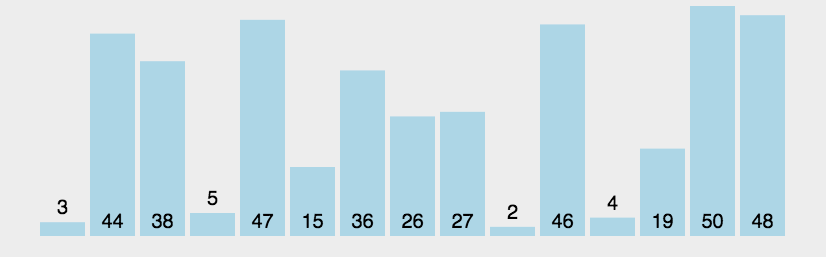冒泡排序的思路是数小的像泡泡一样冒出来，反过来我们可以理解为，数大的像石头一样沉下去。
我们遍历数组，从左到右，若右边的数比左边的大，则交换。
第一遍：最大的数沉下去
第二遍：第二大的数沉在倒数第二个位置

版本二为常见常用版本，版本三在二的基础上优化，避免了不需要的排序

class Sloution {
public:
//冒泡：时间复杂度O(n^2)
//把最小的数放在前面
vector<int> Bubble_Sort(vector<int>& nums) {
int n = nums.size();
for (int i = 0; i < n - 1; i++) {
for (int j = i + 1; j < n; j++) {    //一直把最小的数放在第一位，第二小的数放在第二位，...
if (nums[i] > nums[j]) {
int temp = nums[j];
nums[j] = nums[i];
nums[i] = temp;
}
}
}
return nums;
}

//优化， 以下是最常用的冒泡，，，把最大的数放在后面
//上面的步骤会产生很多无效的排序，比如第一个数是2，最后一个数是1；  交换之后2放到最后面了。 第二次遍历又很麻烦
vector<int> Bubble_Sort02(vector<int>& nums) {
int n = nums.size();
for (int i = 0; i < n - 1; i++) {
for (int j = 0; j < n - i - 1; j++) {
if (nums[j] > nums[j + 1]) {   //修改这里
int temp = nums[j];
nums[j] = nums[j + 1];
nums[j + 1] = temp;
}
}
}
return nums;
}

//优化2
vector<int> Bubble_Sort03(vector<int>& nums) {
int n = nums.size();
bool flag = true;

for (int i = 0; i < n - 1 && flag; i++) {
flag = false;
for (int j = 0; j < n - i - 1; j++) {
if (nums[j] > nums[j + 1]) {   //修改这里
int temp = nums[j];
nums[j] = nums[j + 1];
nums[j + 1] = temp;

flag = true;   //每次循环i有修改，这里为true   如果跑了一次I没有发生交换的情况，说明已经排序完成，不需要再跑后面的i
}
}
}

return nums;
}
}


# （2）选择排序

选择排序：选出最小的数，放在首位； 再次选，放在第二位， …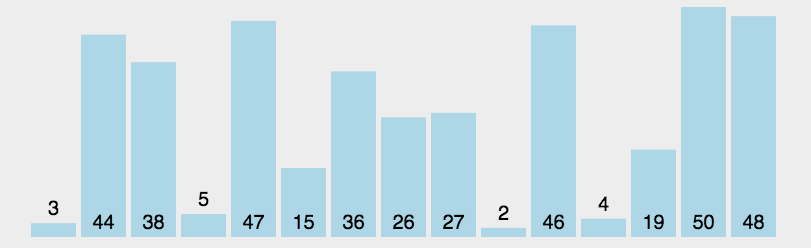//选择排序：选出最小的数，放在首位；  再次选，放在第二位， ...
//时间复杂度：O(n^2)    性能略优于冒泡排序
//类似于在冒泡1基础上改进
vector<int> Simple_Selection_Sort(vector<int>& nums) {
int n = nums.size();
int min;
for (int i = 0; i < n; i++) {
min = i;

for (int j = i + 1; j < n; j++) {
if (nums[j] < nums[min]) {
min = j;
}
}

int temp = nums[i];
nums[i] = nums[min];
nums[min] = temp;
}

return nums;
}


# （3）直接插入排序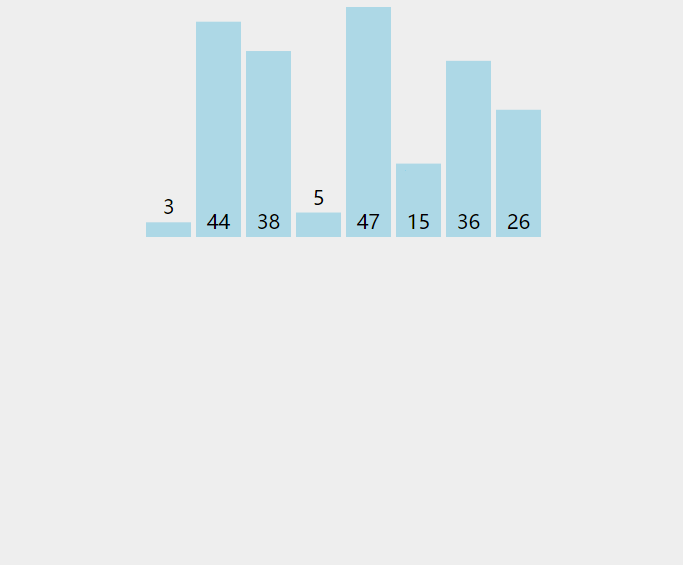vector<int> InsertSort(vector<int>& nums) {
for (int i = 1; i < nums.size(); i++) {   //从索引1开始  往前插入
int temp = nums[i];
int j = i - 1;

for (j; j >= 0 && nums[j] > temp; j--) {   //前面的数  >  后面的数,  实现后移
nums[j + 1] = nums[j];
}
nums[j + 1] = temp;
}

return nums;
}


时间复杂度：
最好的情况：所有数据是正序，每次排序都不用移动元素，此时为O(N)；
最坏的情况：所有数据都是倒序，每次都要将前面的元素后移，此时为O(N^2);

空间复杂度：
在排序的过程中，需要一个临时存储取出值的temp变量，所以空间复杂度为O(1)；

直接插入排序是稳定的排序算法，因为它不需要改变相同元素的位置；

# （4）希尔排序

是在直接插入基础上进行改进，跳着插

	//增量排序
//时间复杂度：O(n^1.5)
vector<int> ShellSort(vector<int>& nums){
int n = nums.size();
int gap = n;  //增量

while (gap > 1){
gap = gap / 3 + 1;   //增量序列

for (int i = gap; i < n; i++){
int temp = nums[i];
int j = i - gap;

for (j; j >= 0 && nums[j] > temp; j = j - gap){  //跳跃式前移
nums[j + gap] = nums[j];
}

nums[j + gap] = temp;
}
}
return nums;
}


# （5）堆排序

是对简单选择排序的改进
参考博客：博客链接，写的很清楚，就是看不懂…
先记录吧

class Sloution02 {
public:
void heapfi(vector<int>& nums, int n, int i)  //对一颗完全二叉树的指定非叶子节点及其叶子结点进行堆的建立
{
//int n = nums.size();
if (i >= n) {  //如果i>=n的时候，证明数组中的元素都已经建立成大根堆了，这是递归终止标志
return;
}
int lchild = i * 2 + 1;    //左孩子节点的下标
int rchild = i * 2 + 2;    //右孩子节点的下标
int max = i;               //默认数值最大的节点为该非叶子节点的值

if (lchild < n && nums[lchild] > nums[max]) {  //判断左孩子节点是否在索引范围内及左孩子结点值是否大于根节点
max = lchild;                              //大于的话就将较大值的下标记录在max中
}
if (rchild < n && nums[rchild] > nums[max]) {   //同上
max = rchild;
}
if (max != i) {
swap(nums[max], nums[i]);   //如果最大值的下标改变，则需要交换两个下标所对应的值
heapfi(nums, n, max);       //对剩下的不是完全二叉树的元素继续进行堆的建立， 套娃
}
}

void build_heap(vector<int>& nums) {
int n = nums.size();
int last_node = n - 1;
int parent = (last_node - 1) / 2;  //从一棵树的最后一个非叶子节点开始建立堆

for (int i = parent; i >= 0; i--)
{
heapfi(nums, n, i);
}
}

//堆排序
vector<int> heap_sort(vector<int>& nums) {
int n = nums.size();
build_heap(nums);                 //先建立一个大根堆

for (int i = n - 1; i >= 0; i--)  //循环交换大根堆中的第一个元素和最后一个元素，并将交换后的最后一个元素出局
{
swap(nums[i], nums);      //交换第一个元素和最后一个元素
heapfi(nums, i, 0);          //调整剩下元素的位置，构建一个新的大根堆，从i号元素开始，即是将交换后的最后一个元素出局
}
return nums;
}

};


# （6）桶排序

1. 准备一个很大的容器，包含所有数出现的范围。比如就假设第一个容器就装0出现的次数，第二个容器装1出现的次数…
2. 初始化容器里面的数都为0
3. 然后遍历待排序的数组，比如数组中有两个0，然后标号为0的桶子就记录0出现的次数，即装2；出现一个5，就在标号为5的桶子里装1
4. 再次遍历容器输出桶子里非零的桶子标号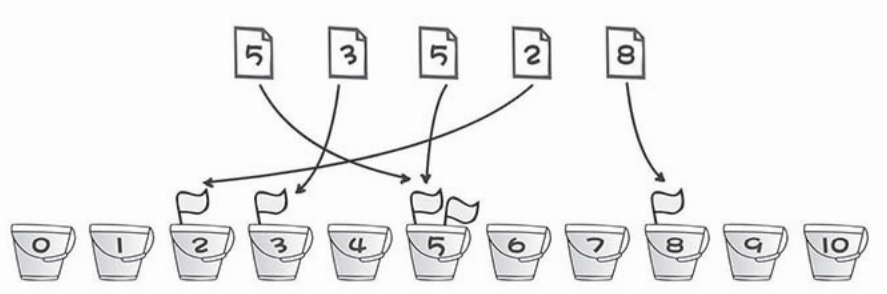挺简单的代码这里就不实现，并没有一个统一的模板，因数组大小不一样。
桶排序浪费空间
适用于没有过多重复元素的数组，时间复杂度大致看为O(n)

# （7）基数排序

参考博客：链接

class Sloution03 {
public:
int MaxBit(vector<int>& input)    //求出数组中最大数的位数
{
int max_num = input;      //默认最大数为第一个数字
for (int i = 0; i < input.size(); i++)  //找出数组中的最大数
{
if (input[i] > max_num)
{
max_num = input[i];
}
}
int p = 0;
while (max_num > 0)
{
p++;
max_num /= 10;   //每次除以10取整，即可去掉最低位
}
return p;
}

int GetNum(int num, int d)   //取出所给数字的第d位数字
{
int p = 1;
while (d - 1 > 0)
{
p *= 10;
d--;
}
return num / p % 10;
}

//基数排序
int n = nums.size();
vector<int> bucket(n);   //创建临时存放排序过程中的数据
vector<int> count(10);   //创建按位计数的技术容器，即记录排序中按个位、十位...各个数的位置的个数

for (int d = 1; d <= MaxBit(nums); d++) {
// 计数器清0
for (int i = 0; i < 10; i++) {
count[i] = 0;
}

// 统计各个桶中的个数
for (int i = 0; i < n; i++) {
count[GetNum(nums[i], d)]++;
}

for (int i = 1; i < 10; i++) {     //得到每个数应该放入bucket中的位置
count[i] += count[i - 1];
}

for (int i = n - 1; i >= 0; i--) {  //采用倒序进行排序是为了不打乱已经排好的顺序
int k = GetNum(nums[i], d);
bucket[count[k] - 1] = nums[i];
count[k]--;
}

for (int j = 0; j < n; j++)    // 临时数组复制到 input 中
{
nums[j] = bucket[j];
}
}
return nums;
}
};


# （8）归并排序

参考博客：链接链接2

该算法是典型的分治策略算法，基本思想是将待排序数组分成若干个小数组，直到为单个数组（即为有序数组）后（分阶段），再将前面得到的单个数组依次拼接在一起，组成有序数组（治阶段）。
动态效果示意图如下：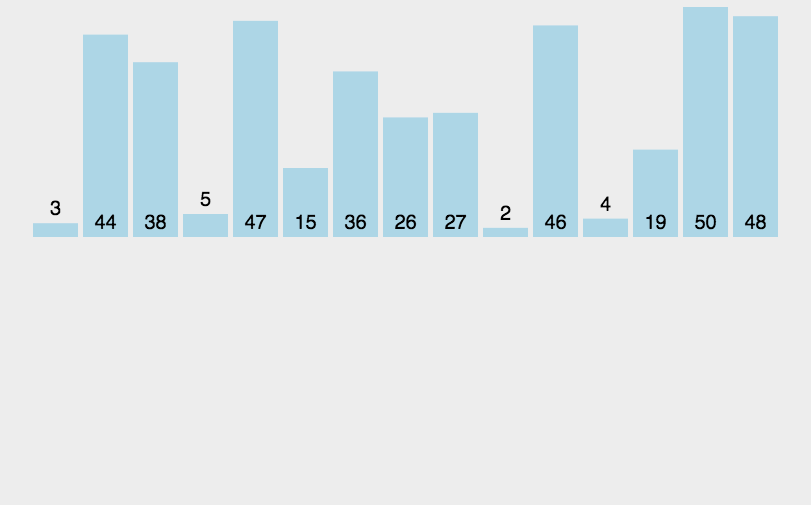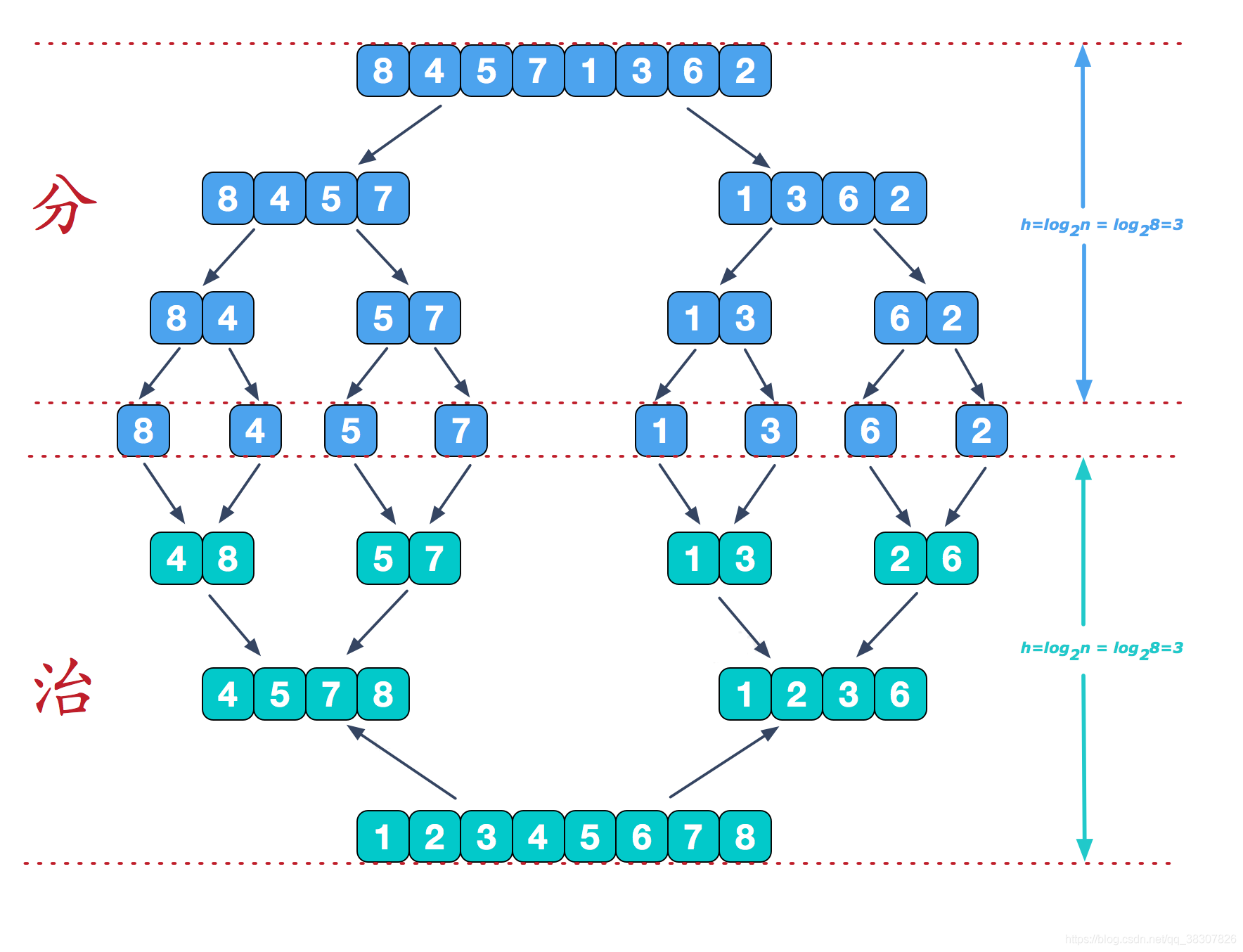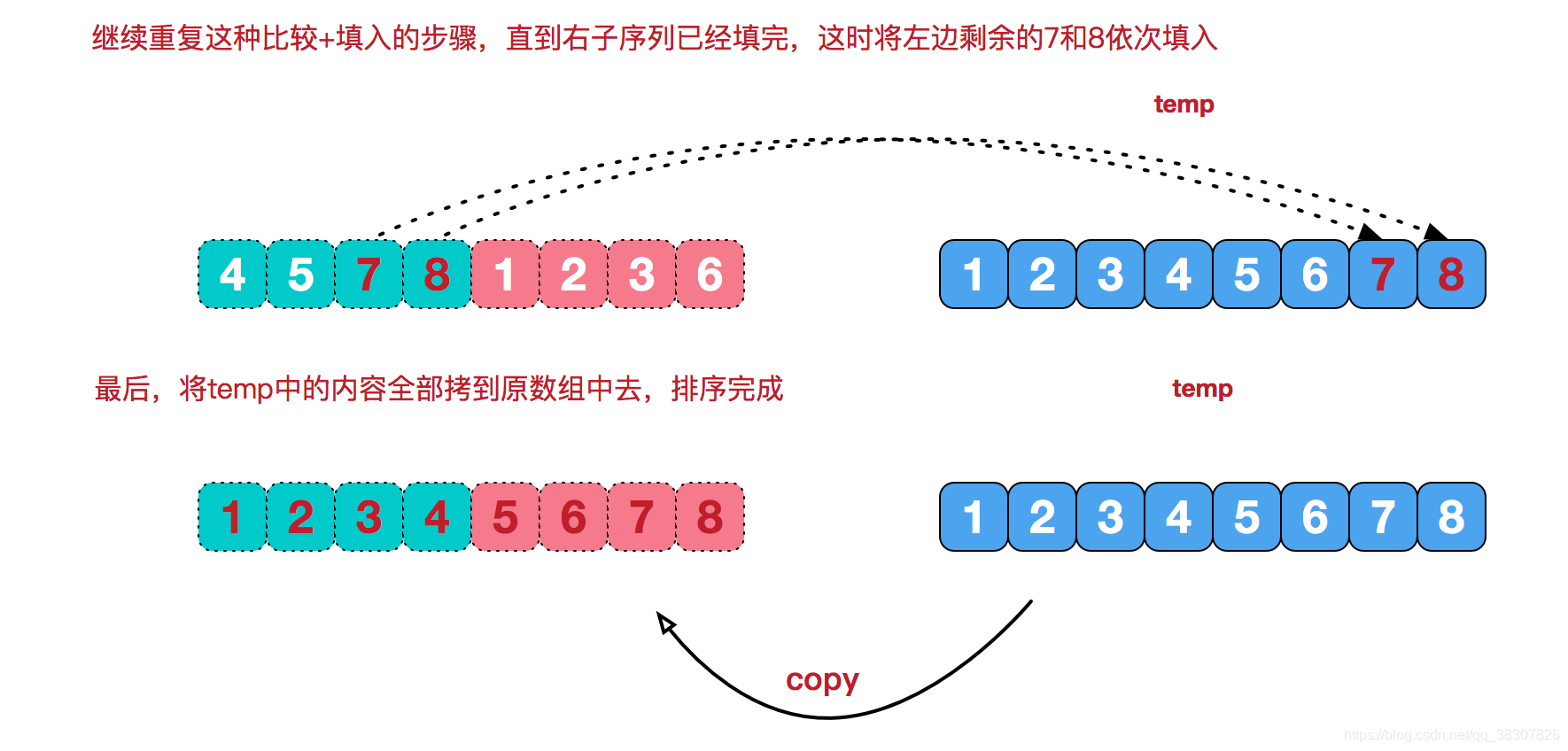#include<iostream>
#include<algorithm>
using namespace std;

void merge(int* data, int start, int end, int* result)
{
int left_length = (end - start + 1) / 2 + 1;
int left_index = start;
int right_index = start + left_length;
int result_index = start;
while (left_index < start + left_length && right_index < end + 1)  //store data into new array
{
if (data[left_index] <= data[right_index])
result[result_index++] = data[left_index++];
else
result[result_index++] = data[right_index++];
}
while (left_index < start + left_length)
result[result_index++] = data[left_index++];
while (right_index < end + 1)
result[result_index++] = data[right_index++];
}

void merge_sort(int* data, int start, int end, int* result)
{
if (1 == end - start)   //last only two elements
{
if (data[start] > data[end])
{
int temp = data[start];
data[start] = data[end];
data[end] = temp;
}
return;
}
else if (end == start)
return; //last one element then there is no need to sort;
else {
//continue to divide the interval
merge_sort(data, start, (end - start + 1) / 2 + start, result);
merge_sort(data, (end - start + 1) / 2 + start + 1, end, result);
//start to merge sorted data
merge(data, start, end, result);
for (int i = start; i <= end; ++i)
{
data[i] = result[i];
}
}

}
//example
int main()
{
int data[] = { 5,3,6,7,3,2,7,9,8,6,34,32,5,4,43,12,37 };
int length = 17;
int result;

merge_sort(data, 0, length - 1, result);
for (int i = 0; i < length; i++)
cout << result[i] << ' ';

system("pause");
return 0;
}

class Sloution02 {
public:
void merge(int arr[], int left, int mid, int right){
int left_size = mid - left;
int right_size = right - mid + 1;
int left_num;
int right_num;
int i, j, k;

//将输入的数组前半部分复制到left_num中
for (i = left; i < mid; i++){
left_num[i - left] = arr[i];
}

//将输入的数组前半部分复制到right_num中
for (i = mid; i <= right; i++){
right_num[i - mid] = arr[i];
}

//将上边的左右数组比较大小后合并到一个数组中
i = 0, j = 0, k = left;
while (i < left_size && j < right_size){
if (left_num[i] < right_num[j])  {//按照从小到大的顺序将元素一次放到arr中
arr[k] = left_num[i];
i++;
k++;
}
else{
arr[k] = right_num[j];
j++;
k++;
}
}

//如果右边部分的数组都放进arr中而左边部分的还有未放入的，就将左边剩余的全部放入
while (i < left_size)   {
arr[k] = left_num[i];
i++;
k++;
}

while (j < right_size){
arr[k] = right_num[j];
j++;
k++;
}

}

void mergeSort(int arr[], int left, int right){
if (left == right){
return;
}
else {
int mid = (left + right) / 2;
mergeSort(arr, left, mid);
mergeSort(arr, mid + 1, right);
merge(arr, left, mid + 1, right);
}
}

//int main()
//{
//	int arr[] = { 2, 5, 4, 3, 9, 1 };
//	int left = 0;
//	int mid = 3;
//	int right = 5;
//	//merge(arr, left, mid, right);
//	mergeSort(arr, left, right);

//	for (int s = 0; s <= right; s++)
//	{
//		cout << arr[s];
//	}
//	system("pause");
//	return 0;
//}

};


二、算法分析：
1、时间复杂度
归并排序的形式就是一棵二叉树，它需要遍历的次数就是二叉树的深度，而根据完全二叉树的可以得出它的时间复杂度是O(n*log2n)。

2、空间复杂度
由前面的算法说明可知，算法处理过程中，需要开辟两个临时数组分别用于存放原属数组的左右两部分。

3、算法稳定性
在归并排序中，相等的元素的顺序不会改变，所以它是稳定的算法

# （9）快速排序

这里可以看书籍《啊哈算法》的讲解，很清楚
采用二分的思想

参考博客：链接

class Sloution04 {
public:
int part(vector<int>& list, int left, int right){
if (list.empty()){
return -1;
}

int base = list[left];  //从最左边的元素为中心元素

while (left < right)   {
while (left < right && list[right] > base)  //判断右侧元素是否大于中心元素，大于就继续遍历
right--;
list[left] = list[right];                   //否则就将右侧小于中心元素的元素挪到左边的位置

while (left < right && list[left] < base)   //判断左侧元素是否小于中心元素，小于就继续遍历
left++;
list[right] = list[left];                   //否则就将左侧小于中心元素的元素挪到右边的位置
}

list[left] = base;                              //最后将中心元素放到左侧位置，此时left左边的元素都比base小
return left;
}

vector<int> QuickSort(vector<int>& list, int left, int right){
if (left < right){
int base = part(list, left, right);  //对数组进行分割，得到分割后的下标

QuickSort(list, left, base - 1);    //左边排序

QuickSort(list, base + 1, right);   //右边排序
}
return list;
}
};

展开全文排序算法
• 排序算法，就是如何使得记录按照要求排列的方法。排序算法在很多领域得到相当地重视，尤其是在大量数据的处理方面。一个优秀的算法可以节省大量的资源。在各个领域中考虑到数据的各种限制和规范，要得到一个符合实际...

# 一、所谓排序，就是使一串记录，按照其中的某个或某些关键字的大小，递增或递减的排列起来的操作。排序算法，就是如何使得记录按照要求排列的方法。排序算法在很多领域得到相当地重视，尤其是在大量数据的处理方面。一个优秀的算法可以节省大量的资源。在各个领域中考虑到数据的各种限制和规范，要得到一个符合实际的优秀算法，得经过大量的推理和分析。

二、排序算法可以分为内部排序外部排序

内部排序是数据记录在内存中进行排序。

外部排序是因排序的数据很大，一次不能容纳全部的排序记录，在排序过程中需要访问外存。

常见的内部排序算法有：冒泡排序选择排序插入排序希尔排序快速排序归并排序等。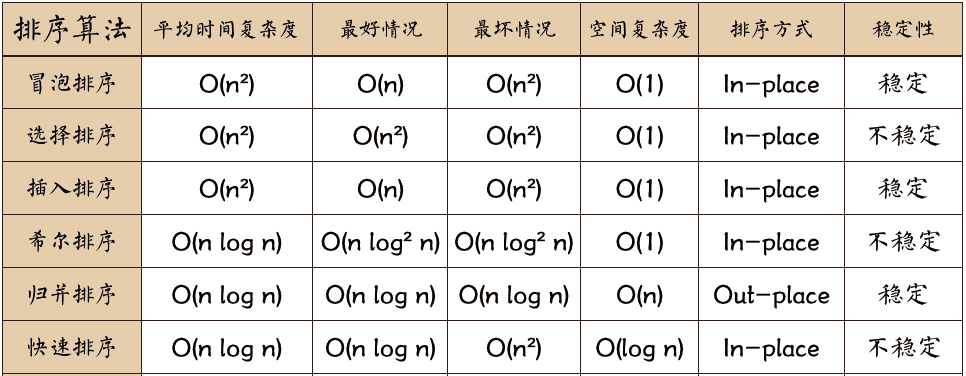三、这里主要介绍常见的几种排序算法

1）冒泡排序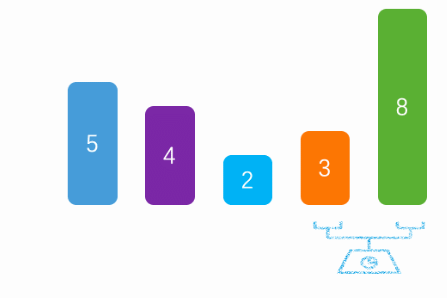a、冒泡排序，是通过每一次遍历获取最大/最小值

b、将最大值/最小值放在尾部/头部

c、然后除开最大值/最小值，剩下的数据在进行遍历获取最大/最小值

d、代码实现public static void main(String[] args) {

int arr[] = {8, 5, 3, 2, 4};

//冒泡
for (int i = 0; i < arr.length; i++) {
//外层循环，遍历次数
for (int j = 0; j < arr.length - i - 1; j++) {
//内层循环，升序（如果前一个值比后一个值大，则交换）
//内层循环一次，获取一个最大值
if (arr[j] > arr[j + 1]) {
int temp = arr[j + 1];
arr[j + 1] = arr[j];
arr[j] = temp;
}
}
}
}e、排序过程（红色：移动的数据）

5,3,2,4,8
3,2,4,5,8
2,3,4,5,8
2,3,4,5,8
2,3,4,5,8

2）选择排序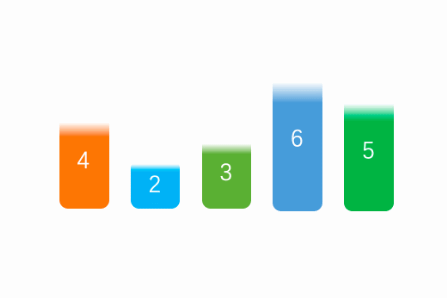a、将第一个值看成最小值

b、然后和后续的比较找出最小值和下标

c、交换本次遍历的起始值和最小值

d、说明：每次遍历的时候，将前面找出的最小值，看成一个有序的列表，后面的看成无序的列表，然后每次遍历无序列表找出最小值。

e、代码实现public static void main(String[] args) {

int arr[] = {6, 5, 3, 2, 4};

//选择
for (int i = 0; i < arr.length; i++) {
//默认第一个是最小的。
int min = arr[i];
//记录最小的下标
int index = i;
//通过与后面的数据进行比较得出，最小值和下标
for (int j = i + 1; j < arr.length; j++) {
if (min > arr[j]) {
min = arr[j];
index = j;
}
}
//然后将最小值与本次循环的，开始值交换
int temp = arr[i];
arr[i] = min;
arr[index] = temp;
//说明：将i前面的数据看成一个排好的队列，i后面的看成一个无序队列。每次只需要找无需的最小值，做替换
}
}f、排序过程（红色：移动的数据）

2,5,3,6,4
2,3,5,6,4
2,3,4,6,5
2,3,4,5,6
2,3,4,5,6

3）插入排序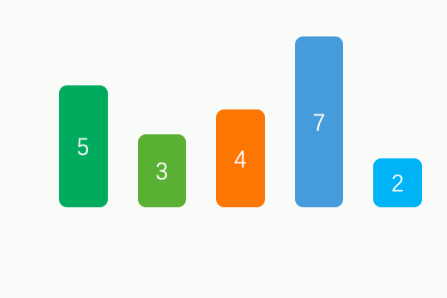a、默认从第二个数据开始比较。

b、如果第二个数据比第一个小，则交换。然后在用第三个数据比较，如果比前面小，则插入（狡猾）。否则，退出循环

c、说明：默认将第一数据看成有序列表，后面无序的列表循环每一个数据，如果比前面的数据小则插入（交换）。否则退出。

d、代码实现public static void main(String[] args) {

int arr[] = {7, 5, 3, 2, 4};

//插入排序
for (int i = 1; i < arr.length; i++) {
//外层循环，从第二个开始比较
for (int j = i; j > 0; j--) {
//内存循环，与前面排好序的数据比较，如果后面的数据小于前面的则交换
if (arr[j] < arr[j - 1]) {
int temp = arr[j - 1];
arr[j - 1] = arr[j];
arr[j] = temp;
} else {
//如果不小于，说明插入完毕，退出内层循环
break;
}
}
}
}e、排序过程（红色：有序，黑色：无序）

5,7,3,2,4
3,5,7,2,4
2,3,5,7,4
2,3,4,5,7

4）希尔排序（插入排序变种版）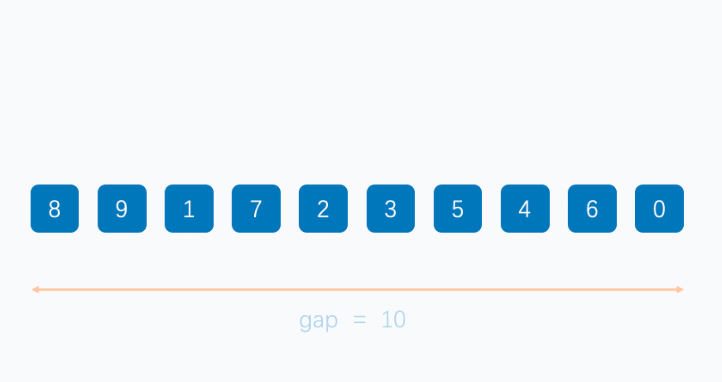a、基本上和插入排序一样的道理

b、不一样的地方在于，每次循环的步长，通过减半的方式来实现

c、说明：基本原理和插入排序类似，不一样的地方在于。通过间隔多个数据来进行插入排序。

d、代码实现public static void main(String[] args) {

int arr[] = {7, 5, 3, 2, 4};

//希尔排序（插入排序变种版）
for (int i = arr.length / 2; i > 0; i /= 2) {
//i层循环控制步长
for (int j = i; j < arr.length; j++) {
//j控制无序端的起始位置
for (int k = j; k > 0  && k - i >= 0; k -= i) {
if (arr[k] < arr[k - i]) {
int temp = arr[k - i];
arr[k - i] = arr[k];
arr[k] = temp;
} else {
break;
}
}
}
//j,k为插入排序，不过步长为i
}
}e、排序过程(步长4/2/1)

4,1,3,2,6,5,8,9,7
3,1,4,2,6,5,7,9,8
1,2,3,4,5,6,7,8,9

5）快速排序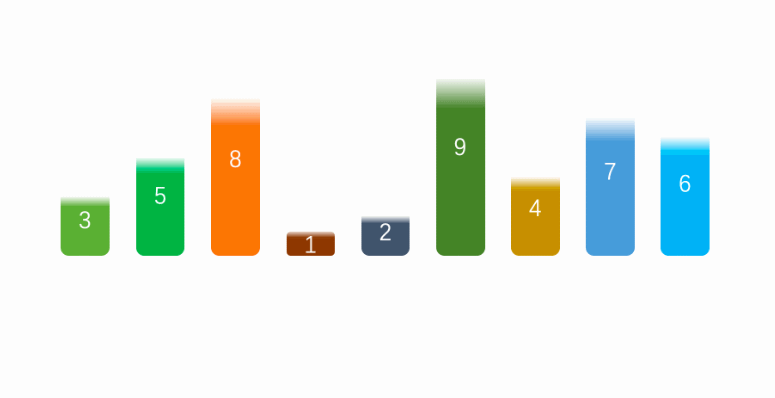a、确认列表第一个数据为中间值，第一个值看成空缺（低指针空缺）。

b、然后在剩下的队列中，看成有左右两个指针（高低）。

c、开始高指针向左移动，如果遇到小于中间值的数据，则将这个数据赋值到低指针空缺，并且将高指针的数据看成空缺值（高指针空缺）。然后先向右移动一下低指针，并且切换低指针移动。

d、当低指针移动到大于中间值的时候，赋值到高指针空缺的地方。然后先高指针向左移动，并且切换高指针移动。重复c、d操作。

e、直到高指针和低指针相等时退出，并且将中间值赋值给对应指针位置。

f、然后将中间值的左右两边看成行的列表，进行快速排序操作。

g、代码实现public static void main(String[] args) {

int arr[] = {7, 5, 3, 2, 4, 1, 8, 9, 6};

//快速排序
int low = 0;
int high = arr.length - 1;
quickSort(arr, low, high);
}

public static void quickSort(int[] arr, int low, int high) {
//如果指针在同一位置(只有一个数据时)，退出
if (high - low < 1) {
return;
}
//标记，从高指针开始，还是低指针（默认高指针）
boolean flag = true;
//记录指针的其实位置
int start = low;
int end = high;
//默认中间值为低指针的第一个值
int midValue = arr[low];
while (true) {
//高指针移动
if (flag) {
//如果列表右方的数据大于中间值，则向左移动
if (arr[high] > midValue) {
high--;
} else if (arr[high] < midValue) {
//如果小于，则覆盖最开始的低指针值，并且移动低指针，标志位改成从低指针开始移动
arr[low] = arr[high];
low++;
flag = false;
}
} else {
//如果低指针数据小于中间值，则低指针向右移动
if (arr[low] < midValue) {
low++;
} else if (arr[low] > midValue) {
//如果低指针的值大于中间值，则覆盖高指针停留时的数据，并向左移动高指针。切换为高指针移动
arr[high] = arr[low];
high--;
flag = true;
}
}
//当两个指针的位置相同时，则找到了中间值的位置，并退出循环
if (low == high) {
arr[low] = midValue;
break;
}
}
//然后出现有，中间值左边的小于中间值。右边的大于中间值。
//然后在对左右两边的列表在进行快速排序
quickSort(arr, start, low -1);
quickSort(arr, low + 1, end);
}h、排序过程(青色：中间值，蓝色：确认位置的数据，红色：移动的数据)6,5,3,2,4,1,7,9,8
1,5,3,2,4,6,7,9,8
1,5,3,2,4,6,7,9,8
1,4,3,2,5,6,7,9,8
1,2,3,4,5,6,7,9,8
1,2,3,4,5,6,7,9,8
1,2,3,4,5,6,7,8,96）归并排序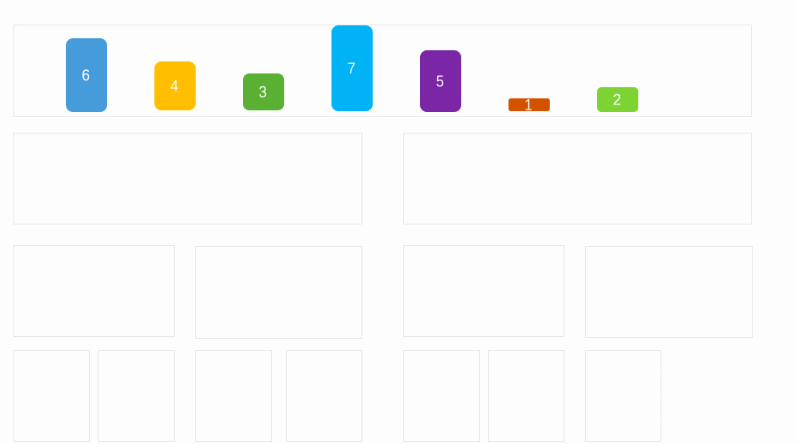a、将列表按照对等的方式进行拆分

b、拆分小最小快的时候，在将最小块按照原来的拆分，进行合并

c、合并的时候，通过左右两块的左边开始比较大小。小的数据放入新的块中

d、说明：简单一点就是先对半拆成最小单位，然后将两半数据合并成一个有序的列表。

e、代码实现public static void main(String[] args) {

int arr[] = {7, 5, 3, 2, 4, 1，6};

//归并排序
int start = 0;
int end = arr.length - 1;
mergeSort(arr, start, end);
}

public static void mergeSort(int[] arr, int start, int end) {
//判断拆分的不为最小单位
if (end - start > 0) {
//再一次拆分，知道拆成一个一个的数据
mergeSort(arr, start, (start + end) / 2);
mergeSort(arr, (start + end) / 2 + 1, end);
//记录开始/结束位置
int left = start;
int right = (start + end) / 2 + 1;
//记录每个小单位的排序结果
int index = 0;
int[] result = new int[end - start + 1];
//如果查分后的两块数据，都还存在
while (left <= (start + end) / 2 && right <= end) {
//比较两块数据的大小，然后赋值，并且移动下标
if (arr[left] <= arr[right]) {
result[index] = arr[left];
left++;
} else {
result[index] = arr[right];
right++;
}
//移动单位记录的下标
index++;
}
//当某一块数据不存在了时
while (left <= (start + end) / 2 || right <= end) {
//直接赋值到记录下标
if (left <= (start + end) / 2) {
result[index] = arr[left];
left++;
} else {
result[index] = arr[right];
right++;
}
index++;
}
//最后将新的数据赋值给原来的列表，并且是对应分块后的下标。
for (int i = start; i <= end; i++) {
arr[i] = result[i - start];
}
}
}f、排序过程（）

5,7,3,2,4,1,6
5,7,2,3,4,1,6
2,3,5,7,4,1,6
2,3,5,7,1,4,6
2,3,5,7,1,4,6
1,2,3,4,5,6,7

四、各种算法的实现方式，肯定有很多种，我这里只要是讲解排序的过程。主要是为了方便理解

展开全文java 数据结构 排序算法 快速排序
• 排序算法的介绍 概述：排序也称排序算法，排序是将一组数据，依指定的顺序进行排列的过程。 排序的分类 (1) 内部排序：指将需要处理的所有数据都加载到内部存储器中进行排序。 (2) 外部排序：数据量过大，无法全部...

# 一. 排序算法的介绍

1. 概述：排序也称排序算法，排序是将一组数据，依指定的顺序进行排列的过程。
2. 排序的分类
(1) 内部排序：指将需要处理的所有数据都加载到内部存储器中进行排序。
(2) 外部排序：数据量过大，无法全部加载到内存中，需要借助外部存储进行排序。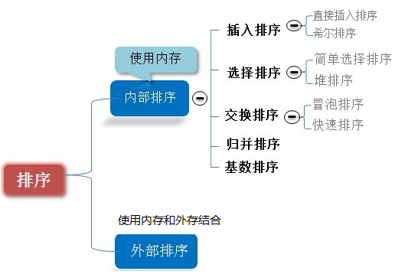# 二. 时间复杂度的相关概念

1. 度量一个程序(算法)执行时间的两种方法。
(1) 事后统计的方法
(2) 事前估算的方法

2. 时间频度的介绍。

 时间频度：一个算法花费的时间与算法中语句的执行次数成正比例，哪个算法中语句执行次数多，它花费时间就多。一个算法中的语句执行次数称为语句频度或时间频度。

3. 时间复杂度的基本介绍。
(1) 一般情况下，算法中的基本操作语句的重复执行次数是问题规模n的某个函数，用T(n)表示，若有某个辅助函数f(n)，使得当n趋近于无穷大时，T(n) / f(n) 的极限值为不等于零的常数，则称f(n)是T(n)的同数量级函数。记作 T(n)=Ｏ( f(n) )，称Ｏ( f(n) ) 为算法的渐进时间复杂度，简称时间复杂度。
(2) T(n) 不同，但时间复杂度可能相同。 如：T(n)=n²+7n+6 与 T(n)=3n²+2n+2 它们的T(n) 不同，但时间复杂度相同，都为O(n²)。

4. 计算时间复杂度的方法。
(1)用常数1代替运行时间中的所有加法常数。 T(n)=n²+7n+6 => T(n)=n²+7n+1
(2)修改后的运行次数函数中，只保留最高阶项。 T(n)=n²+7n+1 => T(n) = n²
(3)去除最高阶项的系数。 T(n) = n² => T(n) = n² => O(n²)

5. 常见的时间复杂度(从小到大排列)。
(1)常数阶O(1)
(2)对数阶O(log2n)
(3)线性阶O(n)
(4)线性对数阶O(nlog2n)
(5)平方阶O(n^2)
(6)立方阶O(n^3)
(7)k次方阶O(n^k)
(8)指数阶O(2^n)

6. 平均时间复杂度和最坏时间复杂度。
(1) 平均时间复杂度是指所有可能的输入实例均以等概率出现的情况下，该算法的运行时间。
(2) 最坏情况下的时间复杂度称为最坏时间复杂度。一般讨论的时间复杂度均是最坏情况下的时间复杂度。 这样做的原因是：最坏情况下的时间复杂度是算法在任何输入实例上运行时间的界限，这就保证了算法的运行时间不会比最坏情况更长。
(3) 平均时间复杂度和最坏时间复杂度是否一致，和算法有关，如下图：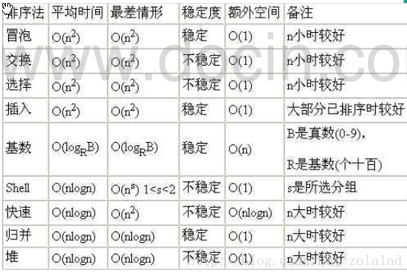# 三. 空间复杂度简介

1. 类似于时间复杂度的讨论，一个算法的空间复杂度定义为该算法所耗费的存储空间，它也是问题规模n的函数。
2. 空间复杂度是对一个算法在运行过程中临时占用存储空间大小的量度。有的算法需要占用的临时工作单元数与解决问题的规模n有关，它随着n的增大而增大，当n较大时，将占用较多的存储单元，例如快速排序和归并排序算法就属于这种情况。
3. 在做算法分析时，主要讨论的是时间复杂度。从用户使用体验上看，更看重的程序执行的速度。一些缓存产品(redis, memcache)和算法(基数排序)本质就是用空间换时间。

# 四. 常用排序算法的时间复杂度对比

1. 对比图如下：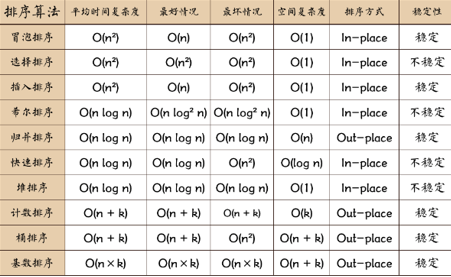2. 相关术语解释。
(1) 稳定：如果a原本在b前面，而a=b，排序之后a仍然在b的前面。
(2) 不稳定：如果a原本在b的前面，而a=b，排序之后a可能会出现在b的后面。
(3) 内排序：所有排序操作都在内存中完成。
(4) 外排序：由于数据太大，因此把数据放在磁盘中，而排序通过磁盘和内存的数据传输才能进行。
(5) 时间复杂度： 一个算法执行所耗费的时间。
(6) 空间复杂度：运行完一个程序所需内存的大小。
(7) n: 数据规模。
(8) k: “桶”的个数。
(9) In-place: 不占用额外内存。
(10) Out-place: 占用额外内存。
展开全文排序算法 数据结构
• 一、冒泡排序 每次冒泡过程都是从数列的第一个元素开始，然后依次和剩余的元素进行比较, 跟列队一样, 从左到右两两相邻的元素比大小, 高的就和低的换一下位置. 最后最高(值最大)的肯定就排到后面了. 但是这只是把...java
• 常见几种排序算法的实现：选择排序、插入排序、冒泡排序、希尔排序、快速排序、归并排序。包括实现与局测试。
• 排序算法(上) https://blog.csdn.net/qq_42453117/article/details/99680831 排序算法(下) https://blog.csdn.net/qq_42453117/article/details/100036347排序算法
• 这是一个非常快的排序方式，V8的sort方法就使用快速排序和插入排序的结合 希尔排序：在非chrome下数组长度小于1000，希尔排序比快速更快 系统方法：在forfox下系统的这个方法非常快 算法源码 代码如下: /
• 种常见排序算法可以分为两大类： 比较类排序： 通过比较来决定元素间的相对次序，由于其时间复杂度不能突破O(nlogn)，因此也称为非线性时间比较类排序。 非比较类排序： 不通过比较来决定元素间的相对次序，它可以...数据结构 排序算法 快速排序
• Dim aData aData = Array(3,2,4,1,6,0) Call ResponseArray(aData, “原来顺序”) Call ResponseArray(SelectSort(aData), “选择排序”) Call ResponseArray(QuickSort(aData), “快速排序”) Call ResponseArray...
• 几种常见的Java排序算法前言一、插入排序二、希尔排序三、冒泡排序四、选择排序五、堆排序六、快速排序七、归并排序 前言 本文介绍了Java中几种常见排序算法 1.插入排序（插入排序，希尔排序） 2.交换排序（冒泡...
• 作者 育人 一实验目的 掌握常见的内部排序算法的思想及其适用条件 掌握常见的内部排序算法的程序实现 二实验内容及要求 任务描述 1任务设计一个内部排序算法模拟系统利用该系统实现常用的7种排序算法并测试各种排序...
• 最近自己总结了js常见几种排序算法，并进行了简单的测试和对比。包括：冒泡排序，插入排序，选择排序，快速排序，归并排序等。 1.冒泡排序： 冒泡排序的原理如同其名，就是先对数组中的n个相邻元素进行两两比较，...排序算法 js算法
• 利用python实现常见几种排序算法，介绍了其实现逻辑和优缺点排序算法 python
• 主要介绍了java几种排序算法的实现及简单分析,实例分析了插入排序、希尔排序、选择排序等常用排序算法,并分析了各个算法的优劣,需要的朋友可以参考下java 排序算法
• 昨天刷面经的时候发现这些基本的算法是面试中常见的问题，吓得我赶紧复习了一下。1.冒泡排序原理：从序列尾至头，依次比较两相邻的元素，小的元素左移（前移）大的元素右移（后移）经过 n-1 次起泡后，序列有序。...排序
• ## 常见的7种排序算法

万次阅读 多人点赞 2018-06-10 11:37:21
最简单的一种排序算法。假设长度为n的数组arr，要按照从小到大排序。则冒泡排序的具体过程可以描述为：首先从数组的第一个元素开始到数组最后一个元素为止，对数组中相邻的两个元素进行比较，如果位于数组左端的元素...选择排序 希尔排序 快速排序 归并排序
• 本文主要记录了几种常见排序算法的Java实现，如冒泡排序、快速排序、直接插入排序、希尔排序、选择排序等等。 在学数据结构与算法时的部分记录，感觉好难啊╮(╯▽╰)╭，还需继续努力。 更多文章欢迎访问我的...数据结构与算法 Java
• 常用的有十种排序算法，包含了插入、选择、交换、分治、线性五种类别，本篇博客将对这十种排序算法做一个总结，并附带C++代码 总体表格 分别来看 插入排序 在要排序的一组数中，假定前n-1个数已经排好序，现在将第n...数据结构 排序 C/C++
• 几种重要的排序算法 1.插入排序 插入排序分为直接插入排序、折半插入排序、希尔排序（shell sort），后两种是在直接插入排序的改进上而来。 1.直接插入排序 排序思路：假设待排序的元素存放在数组A[1..n]A[1..n]A[1...排序算法
• 今天看到了一个算法，感觉以前学过的呢些算法都忘得差不多了，下午复习复习，忘记的上网搜搜，下面的全部编译通过，又学习了点东西。
• ## 常用十大排序算法

千次阅读 2022-04-26 14:39:23
列举了常见的十大内部排序算法：插入排序、希尔排序、选择排序、冒泡排序、归并排序、快速排序、堆排序、基数排序，计数排序，桶排序。排序算法 数据结构
• 常见排序实现起来很简单，不过实际应用很少，复杂度O(n²)。 原理：一趟一趟的比，每一趟中，循环剩余的数，和后一个进行比较，若比它小则交换。这样一趟下来最小的在第一个，最大的在最后一个。总共需要比n-1趟...排序算法 数据结构 java
• 该软件包包含以下常用排序算法的 MATLAB 实现 1) 冒泡排序2）桶排序3) 鸡尾酒排序4) 梳状排序5) 计数排序6) 堆排序7) 插入排序8) 归并排序9) 快速排序10) 基数排序11) 选择排序12) 壳排序 代码的编写方式使得它可以...matlab...